# Electronics and Communication Engineering - Exam Questions Papers

46.

The polar plot for positive frequencies is shown below. The gain margin is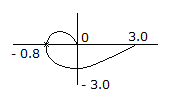A. - 8.02 dB B. 6.02 dB C. 5 dB D. none of these

Answer: Option D

Explanation: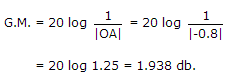47.

Input and output impedance with feedback for voltage series feedback having A = -200, R1 = 10 kΩ, R0 = 50 kΩ and feedback factor β = -0.2 will be

 A. 410 kΩ, 2050 kΩ B. 410 kΩ, 1.219 kΩ C. ≈ 0.243 kΩ, 2050 kΩ D. ≈ 0.24 kΩ, 1.219 kΩ

Answer: Option B

Explanation:

In voltage series feedback output impedance decreases, input impedance increases,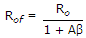, Rif = Ri(1 + Aβ).

48.

Z = __________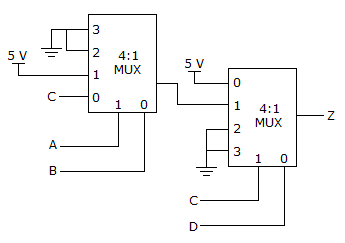A. C[A(B + C) + D] B. (AB + AC + D) C. (CA + AC + D) D. none of these

Answer: Option D

Explanation:

Output of first MUX = A(B + C)

Output of second MUX = C D + A B).

49.

An FM transmitter delivers 80W to a load of 30 W when no modulation is present. The carrier is now frequency modulated by a single sinusoidal signal and the peak frequency deviation is so adjusted to make the amplitude of the second sideband is zero in the given output.
J0(0, 0) = 1,
J0(2, 4) = 0,
J0(3.8) = - 0.4,
J0(5.1)= - 0.1
J1(2, 4) = 0.52,
J1((3.8) = 0,
J1(5.1) = - 0.33,
J2(2.4) = 0.1
J2(3.8)= 0.41,
J2(5.1) = 0
The power in all the remaining sidebands is :

 A. 84 W B. 97.44 W C. 100 W D. 77.44 W

Answer: Option D

Explanation:

Total power of the FM transmitter = 80 W to a load of 50 W

second side band amplitude = J2(β) = 0

β = 5.1

Average Power = (J0(β))2 x 100 = (0.16)2 x 100 = 2.56 W

Power in all remaining = 80 - 2.56 = 77.44 .

50.

In the circuit shown, all the transmission line sections are lossless. The Voltage Standing Wave Ration (VSWR) on the 60W line is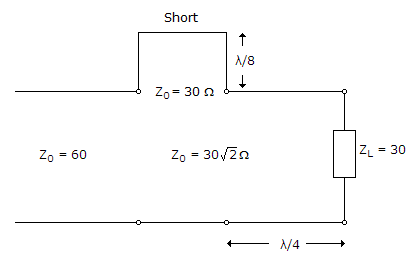A. 1 B. 1.64 C. 2.5 D. 3

Answer: Option B

Explanation: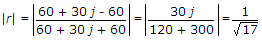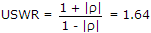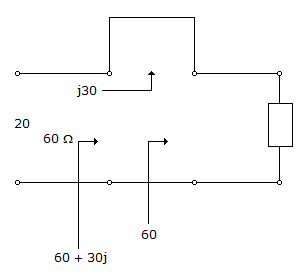#### Current Affairs 2021

Interview Questions and Answers